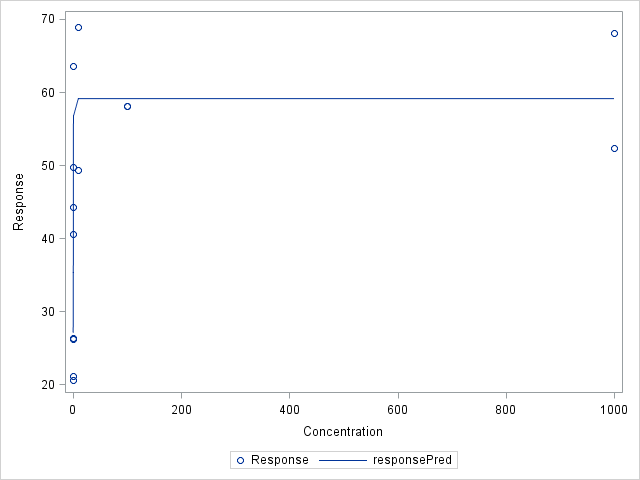## plot nonlinear regression/equation onto a scatter plot

Hi everyone,

I'm a bit embarrassed to ask this, but I'm really not understanding how to plot a nonlinear regression onto a scatter plot.

Currently, I've learned how to use the PROC NLIN procedure in SAS and have been able to generate the same output from an online tutorial: http://www.ats.ucla.edu/stat/sas/faq/doseresponse.htm (yay!).  However, I'm running into a wall when I'm trying to figure out how well the data fits the parameters predicted by SAS.  I want to visually check the predicted variables just in case I need to adjust my starting parameters as indicated in my PARMS procedure.

Here is the following syntax for plotting my data&colon;

PROC IMPORT DATAFILE="C:/Users/jessica/Desktop/SAS/SASUniversityEdition/myfolders/Practice test data.xlsx"
OUT= Work.DoseResp
DBMS=XLSX
REPLACE;
SHEET="DoseResp";

PROC NLIN Data=DoseResp;
PARMS top = 68.875 bottom = 20.575 EC50 = 1 hill = 1;
MODEL response = top + (bottom - top) / (1 + (concentration / EC50)**hill);

data TestNLIN;
x=1;
do i=0 to 1000;
Response = 59.1022 + ( 27.0807 - 59.1022)/(1 + (x/0.8171)**12.4058);
output;
x=x+10;
end;

PROC SGPLOT data = TestNLIN;
scatter x = x y = Response;
yaxis values = (20 to 70 by 10);
xaxis values = (0 to 1000);

PROC SGPLOT data=DoseResp;
Scatter x=Concentration y=response;
yaxis values = (20 to 70 by 10);
xaxis values = (0 to 1000);
RUN;

Does anyone have a better way of plotting a nonlinear regression/plot onto a scatter plot?  Or any other means to visually check how well the data fits to the parameters estimated by SAS?

Much appreciated!

Jess

1 ACCEPTED SOLUTION

Accepted Solutions

## Re: plot nonlinear regression/equation onto a scatter plot

Thank you PG Stats!  That worked perfect!

Just in case someone else runs into the same problem as I did, I thought I would post my syntax.

*Import Excel file*

PROC IMPORT DATAFILE="C:/Users/jessica/Desktop/SAS/SASUniversityEdition/myfolders/Practice test data.xlsx"
OUT= Work.DoseResp
DBMS=XLSX
REPLACE;
SHEET="DoseResp";

*Set Data Set*

DATA pred;
DO concentration = 0 to 1000 by 10;
output;
end;

SET DoseResp;
SET DoseResp pred;
run;

*Use Nonlinear Regression to predict unknow parameters*
PROC NLIN Data=DoseResp;
PARMS top = 68.875 bottom = 20.575 EC50 = 1 hill = 1;
MODEL response = top + (bottom - top) / (1 + (concentration / EC50)**hill);
output out=DRgraph predicted=responsePred;
run;

*Plot data*

proc sort data=DRgraph; by concentration; run;

proc sgplot data=DRgraph;
scatter x=concentration y=response;
series x=concentration y=responsePred;
run;3 REPLIES 3PGStats
Opal | Level 21

## Re: plot nonlinear regression/equation onto a scatter plot

Add some data with missing response values before NLIN so that you get predicted values which you can than plot. Here is an outline:

``````data pred;
do concentration = 0 to 1000 by 10;
output;
end;
run;

data DR;
set DoseResp pred;
run;

PROC NLIN Data=DR;
PARMS top = 68.875 bottom = 20.575 EC50 = 1 hill = 1;
MODEL response = top + (bottom - top) / (1 + (concentration / EC50)**hill);
output out=DRgraph predicted=responsePred;
run;

proc sort data=DRgraph; by concentration; run;

proc sgplot data=DRgraph;
scatter x=concentration y=response;
series x=concentration y=responsePred;
run;``````

(untested)

PG

## Re: plot nonlinear regression/equation onto a scatter plot

Thank you PG Stats!  That worked perfect!

Just in case someone else runs into the same problem as I did, I thought I would post my syntax.

*Import Excel file*

PROC IMPORT DATAFILE="C:/Users/jessica/Desktop/SAS/SASUniversityEdition/myfolders/Practice test data.xlsx"
OUT= Work.DoseResp
DBMS=XLSX
REPLACE;
SHEET="DoseResp";

*Set Data Set*

DATA pred;
DO concentration = 0 to 1000 by 10;
output;
end;

SET DoseResp;
SET DoseResp pred;
run;

*Use Nonlinear Regression to predict unknow parameters*
PROC NLIN Data=DoseResp;
PARMS top = 68.875 bottom = 20.575 EC50 = 1 hill = 1;
MODEL response = top + (bottom - top) / (1 + (concentration / EC50)**hill);
output out=DRgraph predicted=responsePred;
run;

*Plot data*

proc sort data=DRgraph; by concentration; run;

proc sgplot data=DRgraph;
scatter x=concentration y=response;
series x=concentration y=responsePred;
run;## Re: plot nonlinear regression/equation onto a scatter plot

PGStats's solution is a particular case of the general problem of scoring a model on a grid of regularly spaced points. For other options, see  "Techniques for scoring a regression model in SAS."

Discussion stats
• 3 replies
• 3530 views
• 0 likes
• 3 in conversation